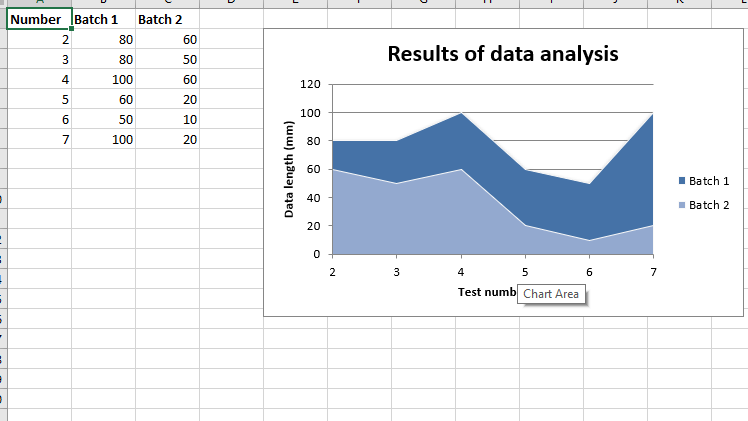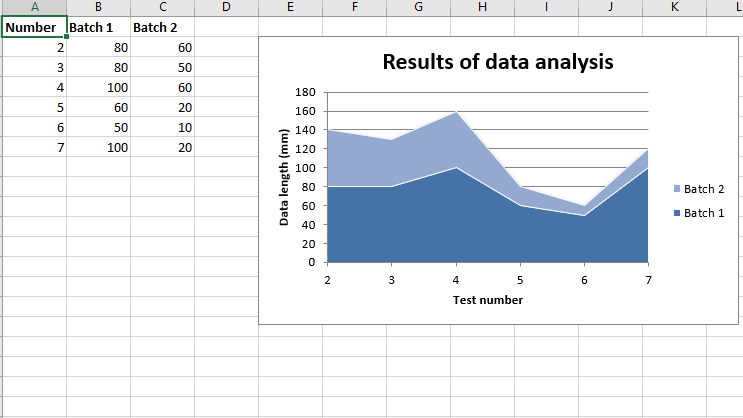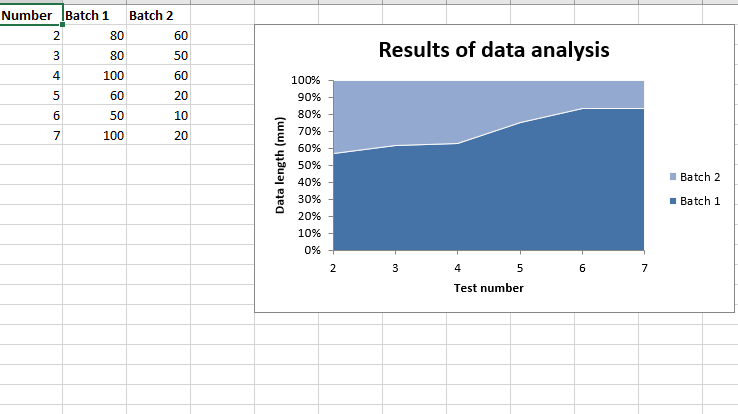# Python | Plotting Area charts in excel sheet using XlsxWriter module

Prerequisite: Creat and write on an excel file

`XlsxWriter `is a Python library using which one can perform multiple operations on excel files like creating, writing, arithmetic operations and plotting graphs. Let’s see how to plot different charts using realtime data.

Charts are composed of at least one series of one or more data points. Series themselves are comprised of references to cell ranges.
For plotting the charts on an excel sheet, firstly, create chart object of specific chart type( i.e Area, Stacked Area, Percent Stacked Area cjhart etc.). After creating chart objects, insert data in it and lastly, add that chart object in the sheet object.

Code #1 : Plot the simple Area Chart.

For plotting the simple Area chart on an excel sheet, use add_chart() method with type ‘area’ keyword argument of a workbook object.

 `# import xlsxwriter module ` `import` `xlsxwriter ` ` `  `# Workbook() takes one, non-optional, argument   ` `# which is the filename that we want to create. ` `workbook ``=` `xlsxwriter.Workbook(``'chart_area.xlsx'``) ` ` `  `# The workbook object is then used to add new   ` `# worksheet via the add_worksheet() method.  ` `worksheet ``=` `workbook.add_worksheet() ` ` `  `# Create a new Format object to formats cells ` `# in worksheets using add_format() method . ` ` `  `# here we create bold format object . ` `bold ``=` `workbook.add_format({``'bold'``: ``1``}) ` ` `  `# create a data list . ` `headings ``=` `[``'Number'``, ``'Batch 1'``, ``'Batch 2'``] ` ` `  `data ``=` `[ ` `    ``[``2``, ``3``, ``4``, ``5``, ``6``, ``7``], ` `    ``[``80``, ``80``, ``100``, ``60``, ``50``, ``100``], ` `    ``[``60``, ``50``, ``60``, ``20``, ``10``, ``20``], ` `] ` ` `  `# Write a row of data starting from 'A1' ` `# with bold format . ` `worksheet.write_row(``'A1'``, headings, bold) ` ` `  `# Write a column of data starting from ` `worksheet.write_column(``'A2'``, data[``0``]) ` `worksheet.write_column(``'B2'``, data[``1``]) ` `worksheet.write_column(``'C2'``, data[``2``]) ` ` `  `# Create a chart object that can be added ` `# to a worksheet using add_chart() method. ` ` `  `# here we create a area chart object . ` `chart1 ``=` `workbook.add_chart({``'type'``: ``'area'``}) ` ` `  `# Add a data series to a chart ` `# using add_series method. ` ` `  `# Configure the first series. ` `# = Sheet1 !\$A\$1 is equivalent to ['Sheet1', 0, 0]. ` `chart1.add_series({ ` `    ``'name'``:       ``'= Sheet1 !\$B\$1'``, ` `    ``'categories'``: ``'= Sheet1 !\$A\$2:\$A\$7'``, ` `    ``'values'``:     ``'= Sheet1 !\$B\$2:\$B\$7'``, ` `}) ` ` `  `# Configure a second series. ` `# Note use of alternative syntax to define ranges. ` `# [sheetname, first_row, first_col, last_row, last_col]. ` `chart1.add_series({ ` `    ``'name'``:       [``'Sheet1'``, ``0``, ``2``], ` `    ``'categories'``: [``'Sheet1'``, ``1``, ``0``, ``6``, ``0``], ` `    ``'values'``:     [``'Sheet1'``, ``1``, ``2``, ``6``, ``2``], ` `}) ` ` `  `# Add a chart title  ` `chart1.set_title ({``'name'``: ``'Results of data analysis'``}) ` ` `  `# Add x-axis label ` `chart1.set_x_axis({``'name'``: ``'Test number'``}) ` ` `  `# Add y-axis label ` `chart1.set_y_axis({``'name'``: ``'Data length (mm)'``}) ` ` `  `# Set an Excel chart style. ` `chart1.set_style(``11``) ` ` `  `# add chart to the worksheet  ` `# the top-left corner of a chart  ` `# is anchored to cell E2 .  ` `worksheet.insert_chart(``'E2'``, chart1) ` ` `  `# Finally, close the Excel file  ` `# via the close() method.  ` `workbook.close() `

Output:Code #2 : Plot the Stacked Area Chart.

For plotting the Stacked Area chart on an excel sheet, use add_chart() method with type ‘area’ and subtype ‘stacked’ keyword argument of a workbook object.

 `# import xlsxwriter module ` `import` `xlsxwriter ` ` `  `# Workbook() takes one, non-optional, argument   ` `# which is the filename that we want to create. ` `workbook ``=` `xlsxwriter.Workbook(``'chart_area2.xlsx'``) ` ` `  `# The workbook object is then used to add new   ` `# worksheet via the add_worksheet() method.  ` `worksheet ``=` `workbook.add_worksheet() ` ` `  `# Create a new Format object to formats cells ` `# in worksheets using add_format() method . ` ` `  `# here we create bold format object . ` `bold ``=` `workbook.add_format({``'bold'``: ``1``}) ` ` `  `# create a data list . ` `headings ``=` `[``'Number'``, ``'Batch 1'``, ``'Batch 2'``] ` ` `  `data ``=` `[ ` `    ``[``2``, ``3``, ``4``, ``5``, ``6``, ``7``], ` `    ``[``80``, ``80``, ``100``, ``60``, ``50``, ``100``], ` `    ``[``60``, ``50``, ``60``, ``20``, ``10``, ``20``], ` `] ` ` `  `# Write a row of data starting from 'A1' ` `# with bold format . ` `worksheet.write_row(``'A1'``, headings, bold) ` ` `  `# Write a column of data starting from ` `worksheet.write_column(``'A2'``, data[``0``]) ` `worksheet.write_column(``'B2'``, data[``1``]) ` `worksheet.write_column(``'C2'``, data[``2``]) ` ` `  `# Create a chart object that can be added ` `# to a worksheet using add_chart() method. ` ` `  `# here we create a stacked area chart object . ` `chart1 ``=` `workbook.add_chart({``'type'``: ``'area'``, ``'subtype'``: ``'stacked'``}) ` ` `  `# Add a data series to a chart ` `# using add_series method. ` ` `  `# Configure the first series. ` `# = Sheet1 !\$A\$1 is equivalent to ['Sheet1', 0, 0]. ` `chart1.add_series({ ` `    ``'name'``:       ``'= Sheet1 !\$B\$1'``, ` `    ``'categories'``: ``'= Sheet1 !\$A\$2:\$A\$7'``, ` `    ``'values'``:     ``'= Sheet1 !\$B\$2:\$B\$7'``, ` `}) ` ` `  `# Configure a second series. ` `# Note use of alternative syntax to define ranges. ` `# [sheetname, first_row, first_col, last_row, last_col]. ` `chart1.add_series({ ` `    ``'name'``:       [``'Sheet1'``, ``0``, ``2``], ` `    ``'categories'``: [``'Sheet1'``, ``1``, ``0``, ``6``, ``0``], ` `    ``'values'``:     [``'Sheet1'``, ``1``, ``2``, ``6``, ``2``], ` `}) ` ` `  `# Add a chart title  ` `chart1.set_title ({``'name'``: ``'Results of data analysis'``}) ` ` `  `# Add x-axis label ` `chart1.set_x_axis({``'name'``: ``'Test number'``}) ` ` `  `# Add y-axis label ` `chart1.set_y_axis({``'name'``: ``'Data length (mm)'``}) ` ` `  `# Set an Excel chart style. ` `chart1.set_style(``11``) ` ` `  `# add chart to the worksheet  ` `# the top-left corner of a chart  ` `# is anchored to cell E2 .  ` `worksheet.insert_chart(``'E2'``, chart1) ` ` `  `# Finally, close the Excel file  ` `# via the close() method.  ` `workbook.close() `

Output:Code #3 : Plot the Percent Stacked Area Chart.

For plotting the Percent Stacked Area chart on an excel sheet, use add_chart() method with type ‘area’ and subtype ‘percent_stacked’ keyword argument of a workbook object.

 `# import xlsxwriter module ` `import` `xlsxwriter ` ` `  `# Workbook() takes one, non-optional, argument   ` `# which is the filename that we want to create. ` `workbook ``=` `xlsxwriter.Workbook(``'chart_area3.xlsx'``) ` ` `  `# The workbook object is then used to add new   ` `# worksheet via the add_worksheet() method.  ` `worksheet ``=` `workbook.add_worksheet() ` ` `  `# Create a new Format object to formats cells ` `# in worksheets using add_format() method . ` ` `  `# here we create bold format object . ` `bold ``=` `workbook.add_format({``'bold'``: ``1``}) ` ` `  `# create a data list . ` `headings ``=` `[``'Number'``, ``'Batch 1'``, ``'Batch 2'``] ` ` `  `data ``=` `[ ` `    ``[``2``, ``3``, ``4``, ``5``, ``6``, ``7``], ` `    ``[``80``, ``80``, ``100``, ``60``, ``50``, ``100``], ` `    ``[``60``, ``50``, ``60``, ``20``, ``10``, ``20``], ` `] ` ` `  `# Write a row of data starting from 'A1' ` `# with bold format . ` `worksheet.write_row(``'A1'``, headings, bold) ` ` `  `# Write a column of data starting from ` `worksheet.write_column(``'A2'``, data[``0``]) ` `worksheet.write_column(``'B2'``, data[``1``]) ` `worksheet.write_column(``'C2'``, data[``2``]) ` ` `  `# Create a chart object that can be added ` `# to a worksheet using add_chart() method. ` ` `  `# here we create a percent stacked area chart object . ` `chart1 ``=` `workbook.add_chart({``'type'``: ``'area'``, ``'subtype'``: ``'percent_stacked'``}) ` ` `  `# Add a data series to a chart ` `# using add_series method. ` ` `  `# Configure the first series. ` `# = Sheet1 !\$A\$1 is equivalent to ['Sheet1', 0, 0]. ` `chart1.add_series({ ` `    ``'name'``:       ``'= Sheet1 !\$B\$1'``, ` `    ``'categories'``: ``'= Sheet1 !\$A\$2:\$A\$7'``, ` `    ``'values'``:     ``'= Sheet1 !\$B\$2:\$B\$7'``, ` `}) ` ` `  `# Configure a second series. ` `# Note use of alternative syntax to define ranges. ` `# [sheetname, first_row, first_col, last_row, last_col]. ` `chart1.add_series({ ` `    ``'name'``:       [``'Sheet1'``, ``0``, ``2``], ` `    ``'categories'``: [``'Sheet1'``, ``1``, ``0``, ``6``, ``0``], ` `    ``'values'``:     [``'Sheet1'``, ``1``, ``2``, ``6``, ``2``], ` `}) ` ` `  `# Add a chart title  ` `chart1.set_title ({``'name'``: ``'Results of data analysis'``}) ` ` `  `# Add x-axis label ` `chart1.set_x_axis({``'name'``: ``'Test number'``}) ` ` `  `# Add y-axis label ` `chart1.set_y_axis({``'name'``: ``'Data length (mm)'``}) ` ` `  `# Set an Excel chart style. ` `chart1.set_style(``11``) ` ` `  `# add chart to the worksheet  ` `# the top-left corner of a chart  ` `# is anchored to cell E2 .  ` `worksheet.insert_chart(``'E2'``, chart1) ` ` `  `# Finally, close the Excel file  ` `# via the close() method.  ` `workbook.close() `

Output:My Personal Notes arrow_drop_upCheck out this Author's contributed articles.

If you like GeeksforGeeks and would like to contribute, you can also write an article using contribute.geeksforgeeks.org or mail your article to contribute@geeksforgeeks.org. See your article appearing on the GeeksforGeeks main page and help other Geeks.

Please Improve this article if you find anything incorrect by clicking on the "Improve Article" button below.

Article Tags :

1

Please write to us at contribute@geeksforgeeks.org to report any issue with the above content.